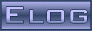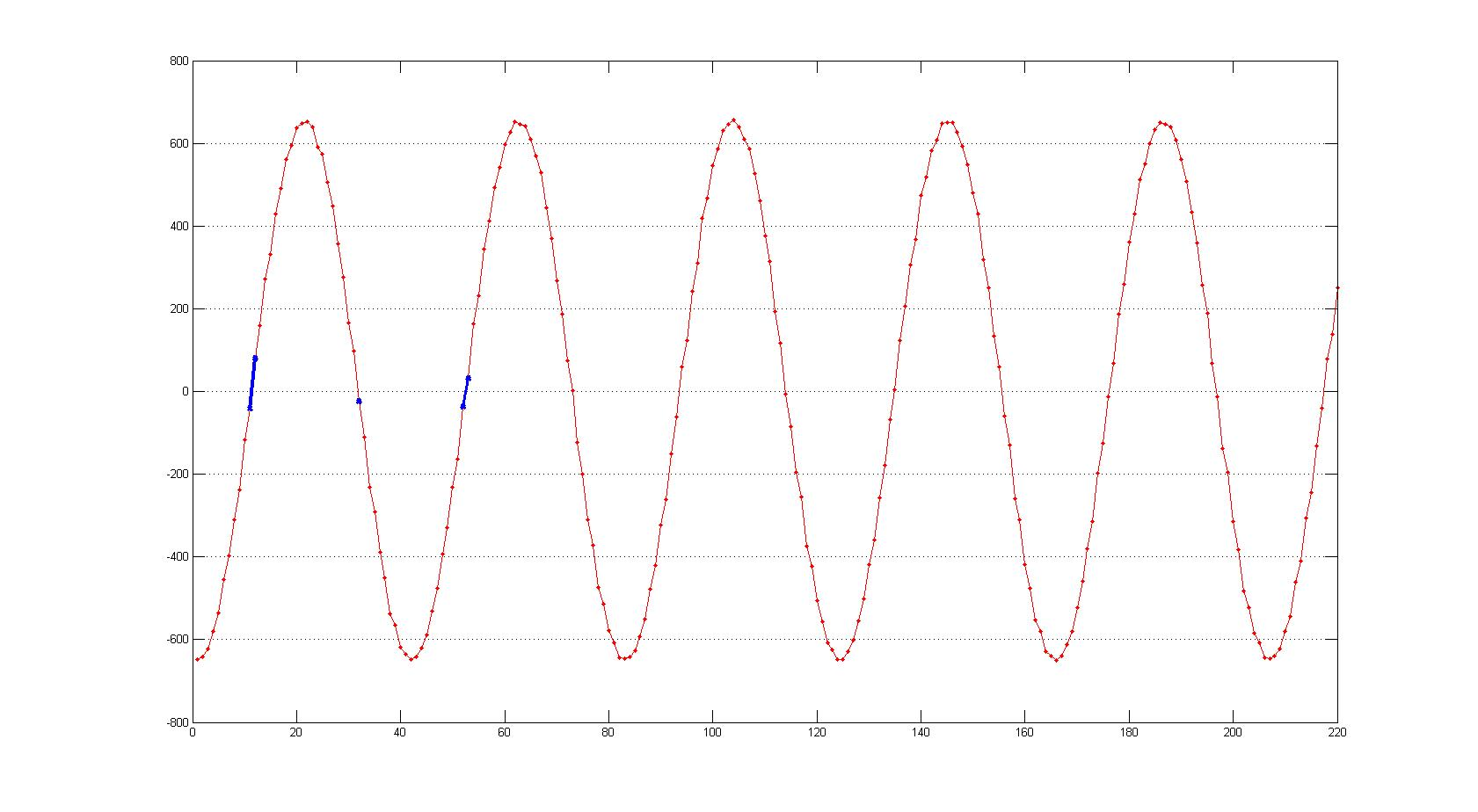DRS4 Forum
 DRS4 Discussion Forum Not logged inMessage ID: 123     Entry time: Tue Jul 12 09:49:08 2011     In reply to: 122     Reply to this: 124
 Author: Jinhong Wang Subject: Fixed Patter Timing Jitter

Stefan Ritt wrote:

Jinhong Wang wrote:

Stefan Ritt wrote:

 Jinhong Wang wrote: Hi Stefan, can you give some suggestions on determination of fixed pattern timing jitter of DRS4?  Thanks~

I will prepare some more detailed description of how to do this in the near future, but we are still learning ourselves constantly how to do that better.

So for the moment I only can recommend you to read the function DRSBoard::CalibrateTiming() and AnalyzeWF(). What you basically do is to define an array of "effective" bin widths t[i]. You start with the nominal bin width (let's say 500ps at 2 GSPS). Now you measure a periodic signal, and look for the zero crossings. If you have a 100 MHz clock, the time between two positive transitions (low-to-high) is 10.000ns. Now you measure the width as seen by the DRS chip, assuming the effective bin widths. The exact zero crossing you interpolate between two samples to improve the accuracy. Now you measure something different, let's say 10.1ns. So you know the ~20 bins between the zero crossings are "too wide", but you don't know which one of them is too wide. So you distribute the "too wide" equally between all bins, that is you decrease the effective width of these bins from 500ps to 500-0.1ns/20=499.995 ps. Then you do this iteratively, that is for all cycles in the waveform, and for many (1000's) of recorded waveforms. It is important that the phase of you measured clock is random, so that all bins are covered equally. Then you realize that the solution oscillates, which you can reduce by using a damping factor (called "damping" in my C code). So you do not correct to 499.995ps, but maybe to 499.999ps. If you iterate often enough, the solution kind of stabilizes.

The attached picture shows the result of such a calibration. Green is the effective bin width which in the end only slightly deviates from 500ps. But the "integral temporal nonlinearity" shows a typical shape for the DRS chip. It's defined as

n
Ti[n] = Sum (t[i]-500ps)
i=0

where t[i] is the effective bin width. So Ti is zero by definition, but the deviation around bin 450 can go up to 1ns at 2 GSPS.

Now you can test you calibration, by measuring again the period of your clock. If you do everything correctly and have a low jitter external clock and no noise on your DRS4 power supply voltages, you should see a residual jitter of about 40ps.

Hope this explanation helps a bit. Let me know if I was not clear enough at some points.

- Stefan

Hi, Stefan,

I noticed other groups of SCA reported the technique to histogram the zero crossings of a sine wave, and use the bin occupancy to derive the effective aperture width.  Recently , I tried this technique to DRS4. In my test, the frequency of the sine wave was selected uncorrelated to the domino frequency.The results were discouraging. Large variations of the domino tap delay was observed.   Besides, I also tried to induce an external trigger, which is uncorrelated to the domino frequency, and histogram the stop positions. Unfortunately, large variations were obtained again. I knew there must be something wrong. Do you have any suggestions?

The attachment is the histogram of the stop positions (the vertical axis is the bin count, the horizontal axis is the bin number). First, I calculate the ration of each bin count to the total counts, supposed the total count is 10000, count of bin 37 is 12, so the corresponding ratio is 12/10000=0.0012. The bin delay is derived by multiplying its ratio to the whole domino period (1024*1/FSamp, eg., for 5 GSP/s, this period is 200ps *1024). (The bin delay i observed was with an variation of about 30 ps).  If the external trigger is uncorrelated to the domino frequency, so, the stop positions are supposed to distribute equally to all bins? If this is true, can i calculate the bin delay via the histogram ?

thank you~

Wang Jinhong

One obvious problem in your method is your statistics. If you have n hits in a bin of the histogram, the error of n is sqrt(n). So if you measure 100 hits, this is more like 100+-10 hits. If you want a better precision, you need much higher statistics. I myself never used this method, but I attach a typical nonlinearity curve running at 2 GSPS, sot hat you know what you should expect. I do some smoothing between neighbor bins so that they do not scatter too much. As you can see, the integral nonlinearity goes almost up to +-2 ns. This value is smaller at higher sampling speeds.

- Stefan

Thank you, Stefan. It is really kind of you to offer suggestions or comments on our concern.

Recently, we input a sine wave to our DRS board. DRS works at about 5 GS/s. The frequency varies from 131 MHz to 231MHz. The attached picture shows the reconstructed points of sine wave (vertical is the amplitude, horizontal axis is the point numbers). We noticed that the variation of the length of the zero crossing segments is very large. The max. length is perhaps two times the length of the min. I marked in blue color in the picture.  It means the corresponding sampling interval of the max. is two times of that of the min.  If this is true, DNL of the DRS sampling interval would be very large. We know, for uniform sampling, the length of the zero crossing segments are assumed to be uniform.  Do you have any comments? Thank you~

 Attachment 1: 131MHz.jpg  101 kB  Uploaded Tue Jul 12 10:49:53 2011ELOG V3.1.4-bcd7b50